# Mechanics of Materials: Stress

Welcome to the Mechanics of Materials. This course builds directly on the fundamentals we learned in Statics – calculating the static equilibrium of various structures under various loads. In statics, we consider the external forces acting on rigid bodies. In reality, all bodies are deformable and those external forces generate internal stresses. Well then, what's a stress?

Stress is the measure of an external force acting over the cross sectional area of an object. Stress has units of force per area: N/m2 (SI) or lb/in2 (US). The SI units are commonly referred to as Pascals, abbreviated Pa. Since the 1 Pa is inconveniently small compared to the stresses most structures experience, we'll often encounter 103 Pa = 1 kPa (kilo Pascal), 106 Pa = a MPa (mega Pascal), or 109 Pa = GPa (giga Pascal).

There are two types of stress that a structure can experience: 1. Normal Stress and 2. Shear Stress. When a force acts perpendicular (or "normal") to the surface of an object, it exerts a normal stress. When a force acts parallel to the surface of an object, it exerts a shear stress.

Let's consider a light fixture hanging from the ceiling by a rope. The cross section of the rope is circular, and the weight of the light is pulling downward, perpendicular to the rope. This force exerts a normal stress within the rope.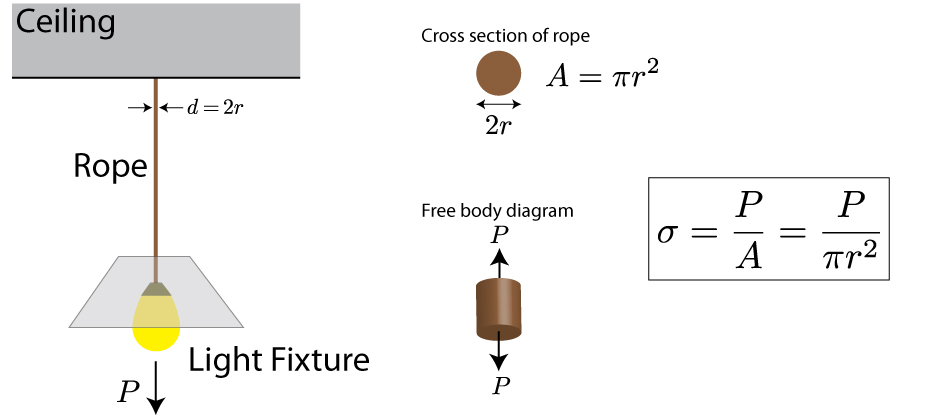Okay, how did we arrive at this equation. There are a lot of assumptions behind the scenes. Throughout this course, we will assume that all materials are homogenous, isotropic, and elastic. We will also assume that the object is "prismatic" – meaning the cross sections are the same all along its length (e.g. a cucumber is prismatic, while a butternut squash is not).  All these assumptions allow us to state that the object will deform uniformly at every point of its cross section. The normal stress at a point on a cross section is defined as (with similar equations in the x and y directions). :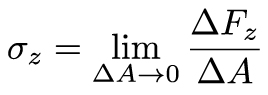Each small area of the cross section is subjected to the same force, and the sum of all these forces must equal the internal resultant force P. If we let ΔA go to dA, and ΔF go to dF, then we can simply integrate both sides of the equation, and we arrive at our relationship for normal stress.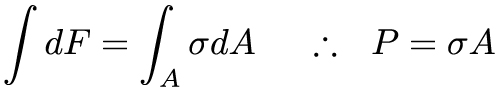This relationship for the normal stress is more accurately an average normal stress, since we've averaged the internal forces over the entire cross section.

Stress is often a difficult concept to grasp because you can’t easily observe it. As it turns out, placing a transparent object through cross polarized light allows you to directly observe stress within a material, based on a concept called photoelasticity:

Stress can actually exist in a material in the absence of an applied load. This is known as residual stress, and it can be used as a way to toughen materials, such as in the fabrication of the Japanese Katana sword. Conversely, undesired residual stresses can encourage crack growth and lead to fracture, such as with the collapse of the Silver Bridge of West Virginia in 1967. Perhaps the most striking example of residual stress is related to the rapid cooling of molten glass, known as “Prince Rupert’s Drop”:

Let's look at another example. Consider a bolt that connects two rectangular plates, and a tensile force perpendicular to the bolt. From a free body diagram, we see that the externally applied force exerts a force parallel to the circular cross section of the bolt. This external force results in a shear stress within the bolt.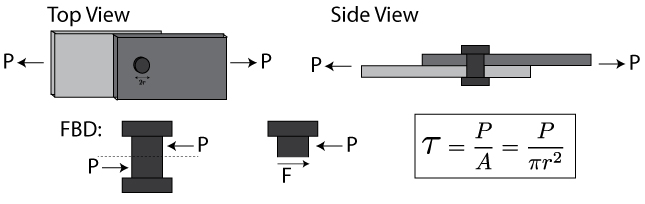Now, the formal definitions of shear stress take on a similar form as those described above. Let's consider the shear stress acting on the z-face of an element: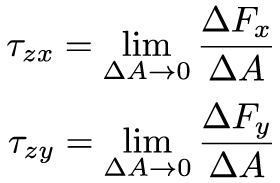The shear stress is the stress acting tangent to the cross section, and it takes on an average value of: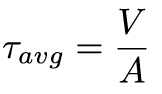It's important to note that the stresses we have just described are average stresses. We have assumed that all of the external force has been evenly distributed over the cross sectional area of the structure – this is not always the case, and we will revisit this assumption throughout the course.

When you look at an element under shear, things seem to be a bit more complicated. Consider a small cubic element within a structure under shear, as shown below.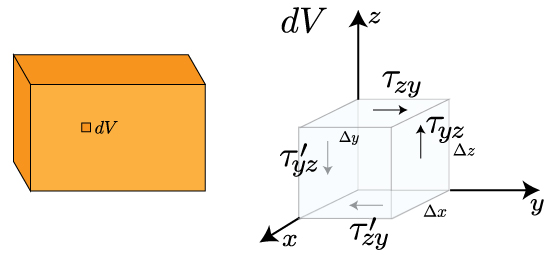Now, equilibrium requires a shear stress acting on τzy to be accompanied by shear stresses on the other planes. But, let's consider the force equilibrium in the y-direction. Knowing that force can be written as stress (tau) times area (ΔxΔy), we can write this force equilibrium as: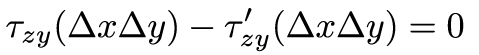Since the areas of the cube are by definition the same, that means τzy = τ'zy.  A similar force equilibrium in the z-direction leads to τyz = τ'yz. Let's consider a moment equilibrium about the x-axis. Knowing that we can write the force as before, and the moment arm will be Δz, this moment balance becomes: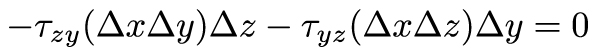This simple relationship tells us that τzy = τyz, and therefore all four shear stresses have equal magnitudes, and must point toward or away from each other at opposite edges of the element. This relationship is known as "pure shear".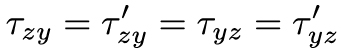### 1.2 Factor of Safety

Engineers use stress to aid in the design of structures. The external load and the geometry of the structure tells us what stress is being exerted within the material, but it tells us nothing about the material itself. Each material has an ultimate stress – a measure of how much stress the material can withstand before failing. To properly design a safe structure, we need to ensure that the applied stress from the external loading never exceeds the ultimate stress of the material.  Part of the difficulty with this task is that we don't always know exactly what the external load is – it may vary unpredictably, and the structure may have to withstand unexpectedly high loads. To account for this uncertainty, we incorporate a Factor of Safety into our design. The factor of safety is just a ratio of the failure load or stress to the allowable load or stress. The failure or ultimate value is a material property while the allowable value is determined by the external force and the geometry of the structure.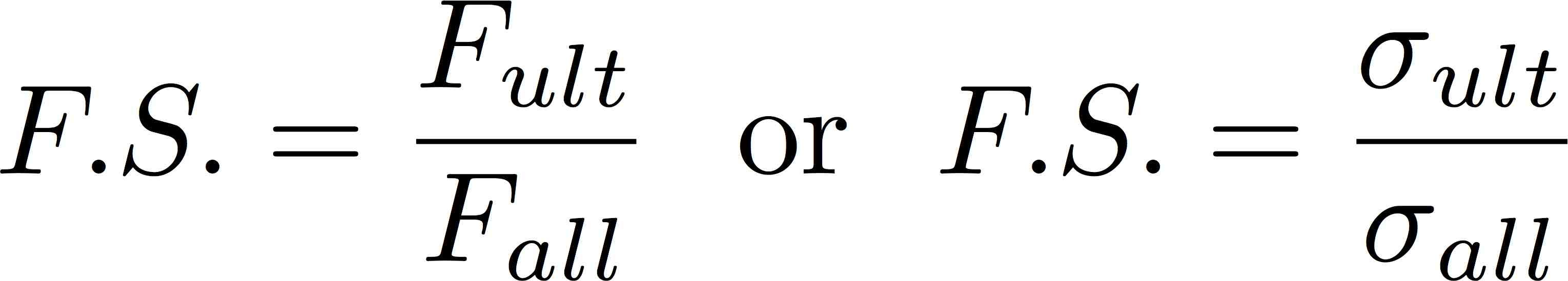## Summary

We've introduced the concept of stress in this lecture. Stress is the a measure of what the material feels from externally applied forces. It is simply a ratio of the external forces to the cross sectional area of the material. Forces that are applied perpendicular to the cross section are normal stresses, while forces applied parallel to the cross section are shear stresses. While the concepts introduced here are not too foreign, much of the difficulty with this material comes from the challenge of calculating the static equilibrium correctly. Calculating static equilibrium will tell us the magnitude and direction of the applied forces, which we can then use to calculate the stresses. If the following example videos and the homework cause you difficulty, now would be a good time to go back and review some of the concepts from your Statics course.This material is based upon work supported by the National Science Foundation under Grant No. 1454153. Any opinions, findings, and conclusions or recommendations expressed in this material are those of the author(s) and do not necessarily reflect the views of the National Science Foundation.